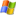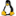Navigation: Home ›  Products ›  Other WaSiM-Helptools ›  Tools for spatial data

# Programs for processing spatial data

 2dsplineResampling of continuous data using bi-cubic spline methods. ascigrid+ gridasciconverting ASCII grid files into binary grid files and vice versa. More » ascii2stackconverting ASCII stacks to binary stacks (single oder double precision). fieldgengeneration of (log-)normal distributed correlated random 2D-arrays as ASCII grid files. More » foclmeanlow pass filter of grid values using a user-defined moving window. gridaddadding two grids cell by cell. gridcolmconverting a binary grid file into an x-y-z format as ASCII file um. The first column contains the x-coordinate, the second column the y-coordinate, and the third column contains the actual cell value. Each line contains such a tripel. The grid header accords to the output of GRIDASCI. griddiffsubtracts the second grid from the first one and writes the result to the output grid. grideditobsolete, use showgrid instead can be used for editing single cells of a binary grid. The coordinates of the cells to be edited must be provided (row and column id's). gridgridMultiplying two grids on y cell-by-cell basis and wrinting the result to the output file. If there is a nodata-value in one of the grids, the result will be nodata as well. gridmaskCreates an output grid by writing the input grids data only for valid cells of an additionally provided mask grid, thus masking the area outside the mask in the output. Cellsizes, number of rows and columns and location of the lower right corner must be identical for both input grids. gridmultMultiplies all cells of a grid with a constant value which will be asked for interactively. Nodata-values in the input grid will not be multiplied with that constant but written as nodata value to the output grid. gridquotDivides all cells of the first input grid with the corresponding cell of the second input grid. Nodata-values in of of the input grid will not be processed but written as nodata value to the output grid. Zero-values in the divisor (2nd grid) may result in NaN-values (not a number). gridstatPrints the statistics of a grid: number of rows and columns, x- and y-coordinates of the lower left corner, cellsize, NODATA-value, cell count (of valid values), sum of all values, minimum and maximum value, average and standard deviation. makegridCreates a grid with a given structure (rows, columns, cellsize, coordinates etc.) and fills it with a constant value (provided as a parameter) for all cells. reclassCreates a classification from the data of the input grid and writes the classes to the output grid (basically by doing an integer division with an optional offset). refinePowerful tool for changing grid resolution and/or coordinates of the lower left corner. Four methods can be used (neares neighbor, two bilinear methods and bicubic spline).More » remapassigning new values to the output grid cells according to the values of a remap table. The remap table defines ranges for the input grid values which are then replaced by a specific value for each such range (one range per row in the format : . resampleCreates an output grid from an input grid with cellsizes that are integer multiples of the input grid cell size. The location (coordinates) of the lower left corner is preserved. There are three methods that could be choosen interactively: 1. arithmetic average, 2. central value, 3. most frequent value. This tools can be used to either resample continous data (e.g. digital elevation model) or to aggregate dicontinous data like land use data (using either the central value or the most frequent value methods). resizeCan be used for cutting a part out of a grid and saving it as the output grid. Useful for clipping a DEM before using it in other tools. The row and column ranges must be provided interactively. stack2gridExtracts a layer from a stack and writes it as result grid. The layer id must be provided interactively. stack2asciiconverting binary stacks (single oder double precision) to ASCII stacks. surfgrid+ gridsurfConverts a SURFERTM ASCII-grid into a binary grid and vice versa topofactComputes the 'soil-topographic index' for each cell, using the flow accumulation, transmissivity and local slope. zonestatCreates a statistic (as ASCII file) of the input grid by using the zones of a zone grid. Each row contains the statistics for all cells of the input grid matching the same zone ID in the zone grid. Statistic values are: zone-ID, zone value, cell count, minimum, maximum, sum, average, standard deviation all toolsContains all previously listed programs for processing spatial data in a single archive# WaSiM-News

05/15/2020

new release availabe:
WaSiM 10.06.00 -> More »